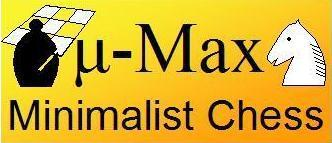```Previous   Next
```

## Evaluation: Piece-Square Tables

#### Positional Points for Pieces in Good Places

To make for at least vaguely sensical positional play in the opening and mid-game, the program needs a clue as to where its pieces are best deployed. A simple way that is in common use to do this are 'piece-square' tables, arrays that for each piece type tabulate the desirability to have that piece on a certain square. In Micro-Max all piece-square tables are condensed into a single one, used for all pieces except Rook and Queen. The latter don't care where the stand, and thus need no table at all. This 'çenter-positional-score' table, since it is indexed by square-number, also occupies blocks of 8 values separated by spaces of 8 unused elements (for the invalid square-numbers). It thus fits nicely in the unused elements of `b[]`, interleaving the game board with the positional table. i.e. the positional score for square x can be found in `b[x+8]`, while the piece on it is found in `b[x]`.

The value to be on a particular square decreases faster if we approach the board edge. This has the effect that in an end-game against a bare King, the engine finds it important to keep that King against the edge or in the corner, even if it has to move his own King slightly off center to achieve this. Unfortunately Kings are also attracted by the center in the middle game...

Below the code lines that implement the piece-square table are highlighted:

```/***************************************************************************/
/*                               micro-Max,                                */
/* A chess program smaller than 2KB (of non-blank source), by H.G. Muller  */
/***************************************************************************/
/* version 3.1 (1997 characters) features:                                 */
/* - recursive negamax search                                              */
/* - quiescence search with recaptures                                     */
/* - recapture extensions                                                  */
/* - (internal) iterative deepening                                        */
/* - best-move-first 'sorting'                                             */
/* - a hash table storing score and best move                              */
/* - full FIDE rules (expt minor ptomotion) and move-legality checking     */

#define F(I,S,N) for(I=S;I<N;I++)
#define W(A) while(A)
#define K(A,B) *(int*)(T+A+(B&8)+S*(B&7))
#define J(A) K(y+A,b[y])-K(x+A,u)-K(H+A,t)

#define U 16777224
struct _ {int K,V;char X,Y,D;} A[U];           /* hash table, 16M+8 entries*/

int V=112,M=136,S=128,I=8e3,C=799,Q,N,i;       /* V=0x70=rank mask, M=0x88 */

char O,K,L,
w[]={0,1,1,3,-1,3,5,9},                        /* relative piece values    */
o[]={-16,-15,-17,0,1,16,0,1,16,15,17,0,14,18,31,33,0, /* step-vector lists */
7,-1,11,6,8,3,6,                          /* 1st dir. in o[] per piece*/
6,3,5,7,4,5,3,6},                         /* initial piece setup      */
b,                                        /* board: half of 16x8+dummy*/
T,                                       /* hash translation table   */

n[]=".?+nkbrq?*?NKBRQ";                        /* piece symbols on printout*/

D(k,q,l,e,J,Z,E,z,n)    /* recursive minimax search, k=moving side, n=depth*/
int k,q,l,e,J,Z,E,z,n;  /* (q,l)=window, e=current eval. score, E=e.p. sqr.*/
{                       /* e=score, z=prev.dest; J,Z=hashkeys; return score*/
int j,r,m,v,d,h,i=9,F,G;
char t,p,u,x,y,X,Y,H,B;
struct _*a=A;
/* lookup pos. in hash table*/
j=(k*E^J)&U-9;                                /* try 8 consec. locations  */
W((h=A[++j].K)&&h-Z&&--i);                    /* first empty or match     */
a+=i?j:0;                                     /* dummy A if miss & full*/
if(a->K)                                      /* hit: pos. is in hash tab */
{d=a->D;v=a->V;X=a->X;                        /* examine stored data      */
if(d>=n)                                     /* if depth sufficient:     */
{if(v>=l|X&S&&v<=q|X&8)return v;             /* use if window compatible */
d=n-1;                                      /* or use as iter. start    */
}X&=~M;Y=a->Y;                               /*      with best-move hint */
Y=d?Y:0;                                     /* don't try best at d=0    */
}else d=X=Y=0;                                /* start iter., no best yet */
N++;                                          /* node count (for timing)  */
W(d++<n|z==8&N<1e7&d<98)                      /* iterative deepening loop */
{x=B=X;                                       /* start scan at prev. best */
Y|=8&Y>>4;                                   /* request try noncastl. 1st*/
m=d>1?-I:e;                                  /* unconsidered:static eval */
do{u=b[x];                                   /* scan board looking for   */
if(u&k)                                     /*  own piece (inefficient!)*/
{r=p=u&7;                                   /* p = piece type (set r>0) */
j=o[p+16];                                 /* first step vector f.piece*/
W(r=p>2&r<0?-r:-o[++j])                    /* loop over directions o[] */
{A:                                        /* resume normal after best */
y=x;F=G=S;                                /* (x,y)=move, (F,G)=castl.R*/
do{H=y+=r;                                /* y traverses ray          */
if(Y&8)H=y=Y&~M;                         /* sneak in prev. best move */
if(y&M)break;                            /* board edge hit           */
if(p<3&y==E)H=y^16;                      /* shift capt.sqr. H if e.p.*/
t=b[H];if(t&k|p<3&!(r&7)!=!t)break;      /* capt. own, bad pawn mode */
i=99*w[t&7];                             /* value of capt. piece t   */
if(i<0||E-S&&b[E]&&y-E<2&E-y<2)m=I;      /* K capt. or bad castling  */
if(m>=l)goto C;                          /* abort on fail high       */

if(h=d-(y!=z))                           /* remaining depth(-recapt.)*/
{v=p<6?b[x+8]-b[y+8]:0;                  /* center positional pts.   */
b[G]=b[H]=b[x]=0;b[y]=u&31;             /* do move, strip virgin-bit*/
if(!(G&M)){b[F]=k+6;v+=30;}             /* castling: put R & score  */
if(p<3)                                 /* pawns:                   */
{v-=9*(((x-2)&M||b[x-2]!=u)+            /* structure, undefended    */
((x+2)&M||b[x+2]!=u)-1);         /*        squares plus bias */
if(y+r+1&S){b[y]|=7;i+=C;}             /* promote p to Q, add score*/
}
v=-D(24-k,-l,m>q?-m:-q,-e-v-i,          /* recursive eval. of reply */
J+J(0),Z+J(8)+G-S,F,y,h);          /* J,Z: hash keys           */
v-=v>e+50;                              /* delayed-gain penalty     */
if(z==9)                                /* called as move-legality  */
{if(v!=-I&x==K&y==L)                    /*   checker: if move found */
{Q=-e-i;O=F;return l;}                 /*   & not in check, signal */
v=m;                                   /* (prevent fail-lows on    */
}                                       /*   K-capt. replies)       */
b[G]=k+38;b[F]=b[y]=0;b[x]=u;b[H]=t;    /* undo move,G can be dummy */
if(Y&8){m=v;Y&=~8;goto A;}              /* best=1st done,redo normal*/
if(v>m){m=v;X=x;Y=y|S&G;}               /* update max, mark with S  */
}                                        /*          if non castling */
t+=p<5;                                  /* fake capt. for nonsliding*/
if(p<3&6*k+(y&V)==S                      /* pawn on 3rd/6th, or      */
||(u&~24)==36&j==7&&                 /* virgin K moving sideways,*/
G&M&&b[G=(x|7)-(r>>1&7)]&32          /* 1st, virgin R in corner G*/
&&!(b[G^1]|b[G^2])                   /* 2 empty sqrs. next to R  */
){F=y;t--;}                              /* unfake capt., enable e.p.*/
}W(!t);                                   /* if not capt. continue ray*/
}}}W((x=x+9&~M)-B);                          /* next sqr. of board, wrap */
C:if(m>I/4|m<-I/4)d=99;                        /* mate is indep. of depth  */
m=m+I?m:-D(24-k,-I,I,0,J,K,S,z,1)/2;         /* best loses K: (stale)mate*/
if(!a->K|(a->X&M)!=M|a->D<=d)                /* if new/better type/depth:*/
{a->K=Z;a->V=m;a->D=d;A->K=0;                /* store in hash,dummy stays*/
a->X=X|8*(m>q)|S*(m<l);a->Y=Y;              /* empty, type (limit/exact)*/
}                                            /*    encoded in X S,8 bits */
/*if(z==8)printf("%2d ply, %9d searched, %6d by (%2x,%2x)\n",d-1,N,m,X,Y&0x77);*/
}
if(z&8){K=X;L=Y&~M;}
return m;
}

main()
{
int j,k=8,*p,c;

for(i=0;i<8;i++)
{b[i]=(b[i+V]=o[i+24]+40)+8;b[i+16]=18;b[i+96]=9;   /* initial board setup*/
for(j=0;j<8;j++)b[16*j+i+8]=(i-4)*(i-4)+(j-3.5)*(j-3.5);   /* center-pts table   */
}                                                   /*(in unused half b[])*/
F(i,M,1035)T[i]=random()>>9;

W(1)                                                /* play loop          */
{F(i,0,121)printf(" %c",i&8&&(i+=7)?10:n[b[i]&15]); /* print board        */
p=c;W((*p++=getchar())>10);                        /* read input line    */
N=0;
if(*c-10){K=c-16*c+C;L=c-16*c+C;}else  /* parse entered move */
D(k,-I,I,Q,1,1,O,8,0);                            /* or think up one    */
F(i,0,U)A[i].K=0;                                  /* clear hash table   */
if(D(k,-I,I,Q,1,1,O,9,2)==I)k^=24;                 /* check legality & do*/
}
}
```
```Previous   Next
```